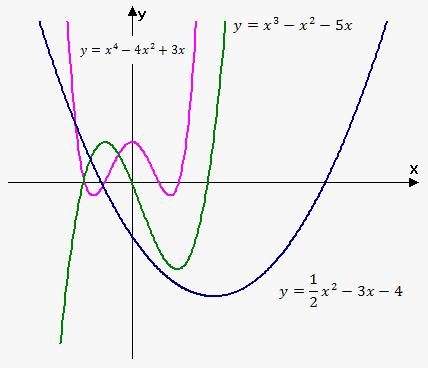# Polynomial Function

Polynomial is a function of the form y=f(x)=a_n x^n+a_(n-1) x^(n-1)+...+a_2 x^2+a_1 x+a_0 , where n is nonnegative integer and a_0,\ a_1,\ ...,\ a_(n-1),\ a_n are constants which are called coefficients of polynomial.

Domain of any polynomial is (-oo,oo).

If a_n!=0 then n is the degree of polynomial. For example, f(x)=-2x^5+3x+sqrt(3) is a polynomial of degree 5.

If degree of polynomial is n=1, then color (blue)(f(x)=mx+b) and it is linear function.

In case degree is 2 then color(red)(f(x)=ax^2+bx+c) and this function is called quadratic.

A polynomial of degree 3 color(green)(f(x)=ax^3+bx^2+cx+d) is called cubic function.

Graph of polynomial functions have different shapes. On the figure you can see graph of polynomials with degree n=2,3,4.